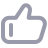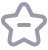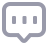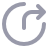# MySQL的前缀索引及Oracle的类似实现

514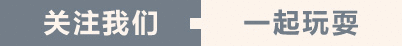MySQL有一个很有意思的索引类型，叫做前缀索引，它可以给某个文本字段的前面部分单独做索引，从而降低索引的大小。

MySQL的前缀索引

MySQL的前缀索引指的是对指定的栏位的前面几位建立的索引。

Createindex index_name on Table_Name(column_name(prefix_len));

Select ..from table_name where column_name=’…’;

delimiter;;

dropFUNCTION if exists random_str;;

CREATEFUNCTION random_str(n int) RETURNS varchar(30000)

begin

declarereturn_str varchar(30000) default "";

declare iint default 0;

whilelength(return_str) < n do

setreturn_str=concat(return_str,md5(rand()));

endwhile;

returnsubstring(return_str,1,n);

end;;

CREATETABLE TEST_PREFIX_IND (

ID INT(10) PRIMARY KEY AUTO_INCREMENT,

NORMAL_STR VARCHAR(20) ,

LONG_STR VARCHAR(1000),

TEXT_STR TEXT,

BLOB_STR BLOB

);

drop procedure if exists init_test_prefix_ind;;

createprocedure init_test_prefix_ind(n int)

begin

declare iint default 0;

while i< n do

insertinto test_prefix_ind(NORMAL_STR,long_str, TEXT_STR,BLOB_STR)

values(random_str(20),random_str(rand()*1000+1),random_str(rand()*1000+1),random_str(rand()*300+1));

seti=i+1;

endwhile;

end;;

callinit_test_prefix_ind(10000);;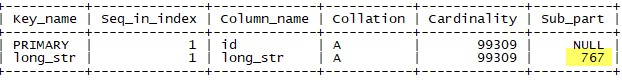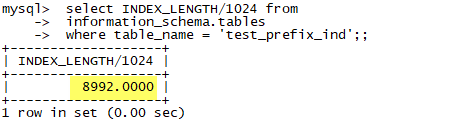mysql> alter table test_prefix_ind add key(text_str);

ERROR 1170 (42000): BLOB/TEXT column 'text_str' used in key specification without a key length

mysql> alter table test_prefix_ind add key(blob_str);;

ERROR 1170 (42000): BLOB/TEXT column 'blob_str' used in key specification without a key length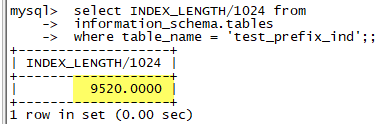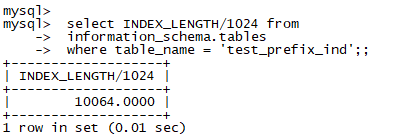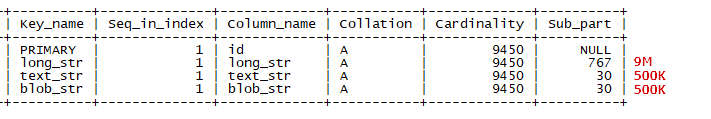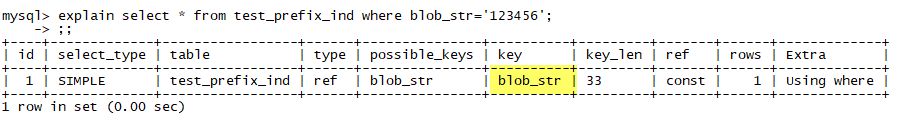Select count(distinct substr(long_str,1,5))/count(*) from test_prefix_ind;

select R,count(distinct substr(long_str,1,R))/count(*)

from

(SELECT @rownum:=ceil(@rownum*1.4) AS R

FROM (SELECT @rownum:=1) r,test_prefix_ind limit 1,10

) R,test_prefix_ind T

group by R;;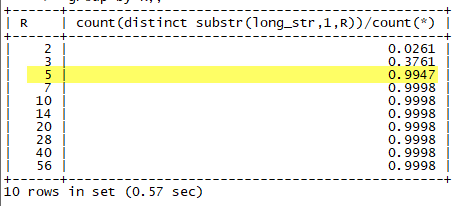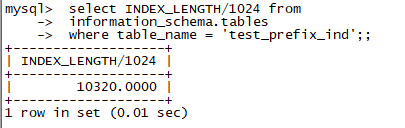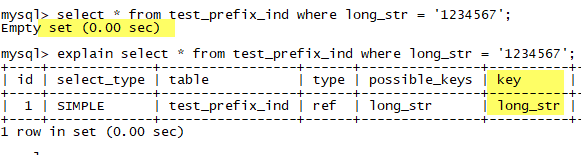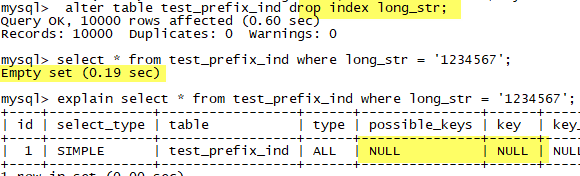Oracle的类似实现

Create index index_name on table_name(substr(column_name,1,) );

Create table test_substr as

select object_id,object_name||dbms_random.string('x',dbms_random.value(1,1000) as object_name,created from all_objects ,

(select * from dual connect by level < 100)

where rownum < 10000;

Create index test_substr_inx on test_substr(substr(object_name,1,5));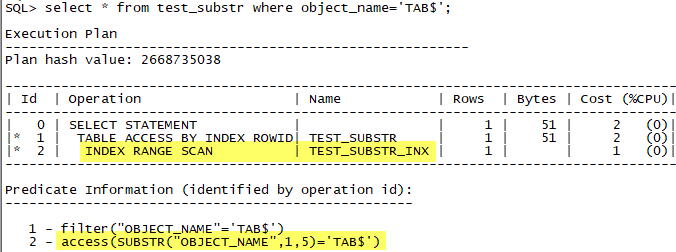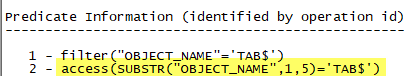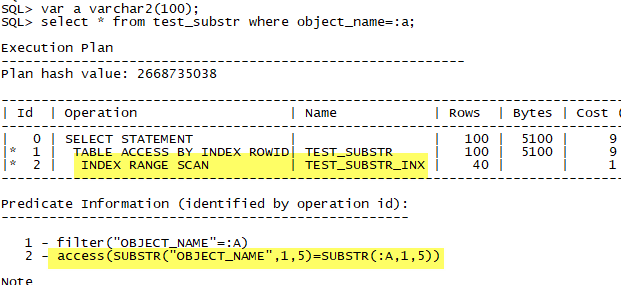select * from test_substr where object_name=:a;

select * from test_substr where object_name=:a and substr(object_name,1,5)=substr(:a,1,5);

SELECT * FROM TEST_SUBSTR WHERE OBJECT_NAME=:A AND SUBSTR(OBJECT_NAME,1,5)=SUBSTR(:A,1,5);

Create index test_substr_inx2 on test_substr(object_name);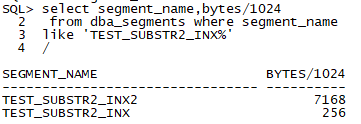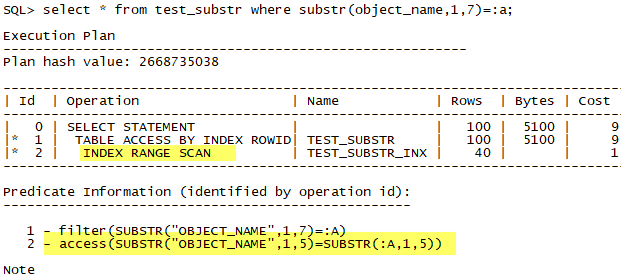select * from test_substr

where substr(object_name,1,)=:a;

select * from test_substr

where substr(object_name,1,)=:a and substr(object_name,1,5)=substr(:a,1,5);

Oracle时间、数字上的前缀索引

Create index test_trunc_date_inx on test_substr(trunc(created));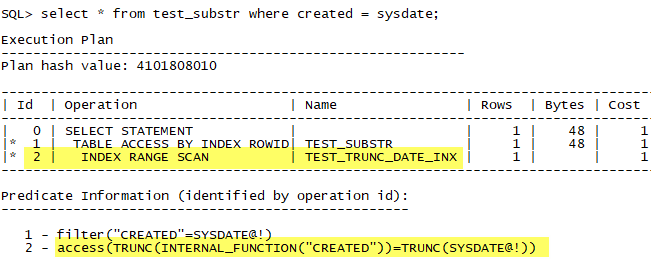create index test_trunc_number on TEST_SUBSTR(trunc(object_id));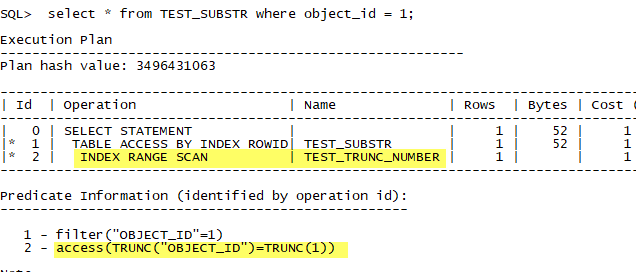create table test_scale (object_name varchar2(5));

insert into test_scale select substr(object_name,1,5) from all_objects;

create index test_scale_str_inx in test_scale(object_name);

select * from test_scale where object_name = 'DBA_TABLES';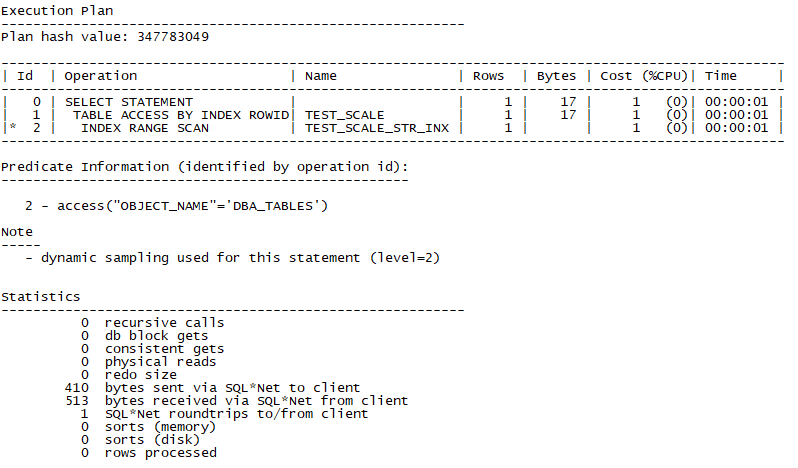‘2017DTC’，2017DTC大会PPT

‘DBALIFE’，“DBA的一天”海报

‘DBA04’，DBA手记4经典篇章电子书

‘RACV1’, RAC系列课程视频及ppt

‘122ARCH’，Oracle 12.2体系结构图

‘2017OOW’，Oracle OpenWorld资料

‘PRELECTION’，大讲堂讲师课程资料